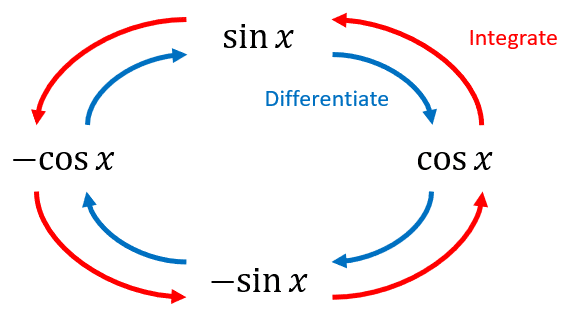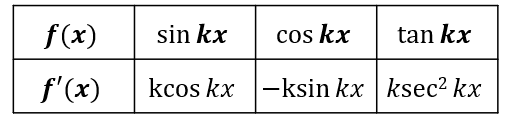# Differentiating Trig Functions

A LevelAQAEdexcelOCR

## Differentiating Trig Functions

We’re now going to look at how to differentiate the simple trig functions – that’s $\textcolor{blue}{\sin}$, $\textcolor{limegreen}{\cos}$ and $\textcolor{red}{\tan}$.

Make sure you are happy with the following topics before continuing.

A LevelAQAEdexcelOCR

## Simple Trig Results

Below is a diagram showing the derivative and integral of the basic trigonometric functions, $\sin x$ and $\cos x$.A LevelAQAEdexcelOCR

## General Trig Differentiation

Below is a table of three derivative results.A LevelAQAEdexcelOCR

## Using the Chain Rule with Trig Functions

We can also use the Chain Rule to differentiate more complex trig functions.

For example, say we have $f(x) = \textcolor{blue}{\sin} (x^2 - x + 1)$.

Then we can set $\textcolor{red}{u} = x^2 - x + 1$ and $f(x) = g(\textcolor{red}{u}) = \textcolor{blue}{\sin} \textcolor{red}{u}$.

Then $\dfrac{d\textcolor{red}{u}}{dx} = 2x - 1$ and $\dfrac{df(x)}{d\textcolor{red}{u}} = \textcolor{limegreen}{\cos} u$.

So,

$\dfrac{df(x)}{dx} = (2x - 1)\textcolor{limegreen}{\cos} (x^2 - x + 1)$

A LevelAQAEdexcelOCR

## From First Principles

We can also find the derivatives from first principles.

For example, let $f(x) = \textcolor{limegreen}{\cos} x$. Then

$f'(x) = \lim\limits_{\textcolor{purple}{h} \to 0}\left( \dfrac{\textcolor{limegreen}{\cos} (x + \textcolor{purple}{h}) - \textcolor{limegreen}{\cos} x}{\textcolor{purple}{h}} \right)$

$= \lim\limits_{\textcolor{purple}{h} \to 0}\left( \dfrac{\textcolor{limegreen}{\cos} x \textcolor{limegreen}{\cos} \textcolor{purple}{h} - \textcolor{blue}{\sin} x \textcolor{blue}{\sin} h - \textcolor{limegreen}{\cos} x}{\textcolor{purple}{h}}\right)$

$= \lim\limits_{\textcolor{purple}{h} \to 0}\left( \dfrac{\textcolor{limegreen}{\cos} x(\textcolor{limegreen}{\cos} \textcolor{purple}{h} - 1) - \textcolor{blue}{\sin} x \textcolor{blue}{\sin} \textcolor{purple}{h}}{\textcolor{purple}{h}}\right)$

Using small angle approximations, we have

$f'(x) = \lim\limits_{\textcolor{purple}{h} \to 0}\left( \dfrac{-\dfrac{1}{2}\textcolor{purple}{h}^2 \textcolor{limegreen}{\cos} x - \textcolor{purple}{h}\textcolor{blue}{\sin} x}{\textcolor{purple}{h}}\right)$

$= \lim\limits_{\textcolor{purple}{h} \to 0}\left( \dfrac{-1}{2}\textcolor{purple}{h}\textcolor{limegreen}{\cos} x - \textcolor{blue}{\sin} x\right)$

$= -\textcolor{blue}{\sin} x$

A LevelAQAEdexcelOCR

## Differentiating Trig Functions Example Questions

$x = \tan y$ gives

$\dfrac{dx}{dy} = \sec ^2 y$

Then $\dfrac{dy}{dx} = \cos ^2 y$.

Let $u = x^2$ and $y = \tan u$.

Then

$\dfrac{dy}{dx} = 2x \times \sec ^2 u = \dfrac{2x}{\cos ^2 x^2}$

$f'(x) = \lim\limits_{h \to 0}\left( \dfrac{\sin (kx + kh) - \sin kx}{h} \right)$

$= \lim\limits_{h \to 0}\left( \dfrac{\sin kx \cos kh + \sin kh \cos kx - \sin kx}{h}\right)$

$= \lim\limits_{h \to 0}\left( \dfrac{\sin kx(\cos kh - 1) + \sin kh \cos kx}{h}\right)$

Using small angle approximations, we have

$f'(x) = \lim\limits_{h \to 0}\left( \dfrac{-\dfrac{1}{2}(kh)^2 \sin kx + kh\cos kx}{h}\right)$

$= \lim\limits_{h \to 0}\left( \dfrac{-1}{2}kh\sin kx + k\cos kx\right)$

$= k\cos kx$

A Level

A Level

A Level

## You May Also Like...### MME Learning Portal

Online exams, practice questions and revision videos for every GCSE level 9-1 topic! No fees, no trial period, just totally free access to the UK’s best GCSE maths revision platform.

£0.00

A Level

A Level